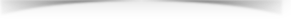﻿ Do You Know How the Vacuum Meter Reading of the Vacuum Drying Oven is Converted to the Vacuum Rate(Pa)? - Guangdong Yuanyao Test Equipment Co.,Ltd.
+86-13532971605• EN

# Do You Know How the Vacuum Meter Reading of the Vacuum Drying Oven is Converted to the Vacuum Rate(Pa)?

Vacuum drying oven makes use of vacuum pump to pump air and humidity out, making the working chamber vacuum and speeding up drying with boiling point depression. It has the advantages of fast drying speed, low pollution, and no damage to the internal quality of the dried goods. But do you know how to convert the reading of vacuum gauge on vacuum oven to the vacuum rate(Pa)? Next, the article will share the conversion method.

(1) "0" on the vacuum gauge represents the standard atmospheric pressure and "-0.1" means complete vacuum. The indicated value on the vacuum gauge is not the complete value of vacuum rate but only the relative value.

(2) The conversion of vacuum rate. According to the scale value range in this table, the complete value of vacuum degree and relative value can be converted by the following formula: P=1×105(1-δ/0.1)P is the complete value of vacuum rate (Pa).

δ-The complete scale value shown on the vacuum gauge.

Example 1: The value shown in the table is 0, P=1×105(1-0/0.1)=1×105 Pa =1 atmospheric pressure

Example 2: The value shown in the table is 0.1, P=1×105(1-0.1/0.1)= 0 Pa is a complete vacuum.

(A complete vacuum does not exist)

Example 3: The value shown in the table is 0.08, so P=1×105(1-0.08/0.1)=2×104Pa

The vacuum indicated value of the product is < 267Pa, indicating that the product can still work regularly under the low vacuum condition at 267Pa (external value is about 0.0997, nearly -0.1). The vacuum rate of the product is mainly determined by the performance of forming a set of pumps.

The conversion of the measurement unit of vacuum rate is as follows:

0.1Mpa =1×105 Pa = 760mmHg =1 atmospheric pressure

1 torr = 1mmHg =133.33Pa

2 torr = 0.00026666 Mpa≈267 Pa

(2) The vacuum gauge readings can be revealed by intuitive numbers. However, the vacuum drying oven price is extremely high and generally suitable for scientific research units.

The above shows the conversion method between vacuum meter reading and vacuum rate(Pa) of vacuum drying chamber.

Environmental Test Equipment recommendation
•••Resources
•Products• +86-13532971605

•• +86-13826920939